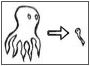ModelReduction.rudnyi.ru

 Home Model Reduction Applications Software
 MOR Home Linear Second Order Parametric Weakly Nonlinear Nonlinear

# Weakly-Nonlinear Model Reduction

When nonlinearity is limited to quadratic and cubic terms only, one can generalize moment matching to treat these terms. This is quite promising for finite element models with weak nonlinearity in material properties.

L. H. Feng, E. B. Rudnyi, J. G. Korvink, C. Bohm, T. Hauck.
Compact Electro-thermal Model of Semiconductor Device with Nonlinear Convection Coefficient.
In: Thermal, Mechanical and Multi-Physics Simulation and Experiments in Micro-Electronics and Micro-Systems. Proceedings of EuroSimE 2005, Berlin, Germany, April 18-20, 2005, p. 372-375.
Final paper at IEEE

Compact thermal models for semiconductor devices are usually constructed under the assumption of constant material properties as well as the convection coefficient. However, in many cases this is the cause of undesired deviations from experimental results. In this paper, we present an extension of model order reduction to construct a compact thermal model for the case when a convection coefficient is nonlinear. As an example, we use transient thermal simulation of a semiconductor device with a temperature-dependent convection coefficient. We demonstrate that the model order reduction technique introduced in this paper can treat such nonlinearity very efficiently. At the same time, the reduced model is accurate enough to replace the original model.

J. R. Phillips.
Projection-based approaches for model reduction of weakly nonlinear, time-varying systems.
IEEE Transactions on Computer-Aided Design of Integrated Circuits and Systems, vol. 22, pp. 171-187, 2003.
Paper at IEEEXplore.

R. D. Slone, R. Lee and J. F. Lee.
Broadband model order reduction of polynomial matrix equations using single point well-conditioned asymptotic waveform evaluation: derivations and theory.
Int. J. Numer. Meth. Engng, vol. 58, no. 15, pp. 2325-2342, 2003.
Paper at InterScience.

J. H. Chen, S. M. Kang, J. Zou, C. Liu, and J. E. Schutt-Aine.
Reduced-order Modeling of weakly Nonlinear MEMS devices with Taylor-Series expansion and Arnoldi approach.
Journal of Microelectromechanical Systems, vol. 13, pp. 441-451, 2004.
Paper at IEEEXplore.

Zhaojun Bai and Daniel Skoogh.
A projection method for model reduction of bilinear dynamical systems.
Linear Algebra and its Applications, v. 415, N 2-3, p. 406-425.
Paper at ScienceDirect.

Lihong Feng.
Review of model order reduction methods for numerical simulation of nonlinear circuits.
Applied Mathematics and Computation 2005, v. 167, N 1, p. 576-591.
Paper at ScienceDirect.